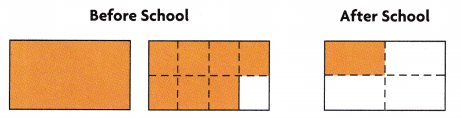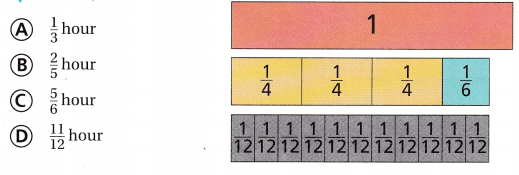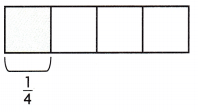Refer to our Texas Go Math Grade 5 Answer Key Pdf to score good marks in the exams. Test yourself by practicing the problems from Texas Go Math Grade 5 Unit 2 Assessment Answer Key.

Vocabulary

Choose the best term from the box.

Vocabulary
common denominator
common multiple

Question 1.
A ___________ is a number that is a multiple of two or more numbers. (p.213)
Answer: A common multiple is a number that is a multiple of two or more numbers.

Concepts and Skills

Use a common denominator to write an equivalent fraction for each fraction. (TEKS 5.3)

Question 2.
$$\frac{2}{5}$$, $$\frac{1}{8}$$ common
denominator: ______________
Given fractions, $$\frac{2}{5}$$, $$\frac{1}{8}$$
The denominators and numerators are different here.
$$\frac{2}{5}$$ × $$\frac{8}{8}$$ = $$\frac{16}{40}$$
$$\frac{1}{8}$$ × $$\frac{5}{5}$$ = $$\frac{5}{40}$$
Thus the equivalent fractions are $$\frac{16}{40}$$, $$\frac{5}{40}$$

Question 3.
$$\frac{3}{4}$$, $$\frac{1}{2}$$ common
denominator: _______________
Given fractions, $$\frac{3}{4}$$, $$\frac{1}{2}$$
The denominators and numerators are different here.
$$\frac{3}{4}$$ × $$\frac{2}{2}$$ = $$\frac{6}{8}$$
$$\frac{1}{4}$$ × $$\frac{2}{2}$$ = $$\frac{2}{8}$$
Thus the equivalent fractions are $$\frac{6}{8}$$ and $$\frac{2}{8}$$

Question 4.
$$\frac{2}{3}$$, $$\frac{1}{6}$$ common
denominator: _____________
Given fractions, $$\frac{2}{3}$$, $$\frac{1}{6}$$
The denominators and numerators are different here.
$$\frac{2}{3}$$ × $$\frac{2}{2}$$ = $$\frac{4}{6}$$
$$\frac{1}{6}$$ × $$\frac{1}{1}$$ = $$\frac{1}{6}$$
Thus the equivalent fractions are $$\frac{4}{6}$$ and $$\frac{1}{6}$$

Find the sum or difference. Write your answer in simplest form. (TEKS 5.3.K)

Question 5.
$$\frac{5}{8}$$ + $$\frac{5}{8}$$
Given,
$$\frac{5}{8}$$ + $$\frac{5}{8}$$
The denominators of both the fractions are same.
$$\frac{5}{8}$$ + $$\frac{5}{8}$$ = (5 + 5)/8 = $$\frac{10}{8}$$ = $$\frac{5}{4}$$ = 1 $$\frac{1}{4}$$

Question 6.
3$$\frac{2}{3}$$ – 1$$\frac{2}{5}$$
3 + $$\frac{2}{3}$$ – 1 – $$\frac{2}{5}$$
3 – 1 = 2
$$\frac{2}{3}$$ – $$\frac{2}{5}$$
$$\frac{2}{3}$$ × $$\frac{5}{5}$$ – $$\frac{2}{5}$$ × $$\frac{3}{3}$$
= $$\frac{10}{15}$$ – $$\frac{6}{15}$$ = $$\frac{4}{15}$$
Thus, 3$$\frac{2}{3}$$ – 1$$\frac{2}{5}$$ = 2$$\frac{4}{15}$$

Question 7.
7$$\frac{3}{5}$$ + 3$$\frac{9}{20}$$
Given,
7$$\frac{3}{5}$$ + 3$$\frac{9}{20}$$
7+ $$\frac{3}{5}$$ + 3 + $$\frac{9}{20}$$
7 + 3 = 10
$$\frac{3}{5}$$ + $$\frac{9}{20}$$
$$\frac{12}{20}$$ + $$\frac{9}{20}$$ = $$\frac{21}{20}$$ = 1 $$\frac{1}{20}$$
10 + 1 + $$\frac{1}{20}$$ = 11$$\frac{1}{20}$$

Find the product. Write the product in simplest form. Use a model. (TEKS 5.3.I)

Question 8.
$$\frac{3}{5}$$ × 8 = ____________
$$\frac{3}{5}$$ × 8 = $$\frac{24}{5}$$ = 4 $$\frac{4}{5}$$

Question 9.
$$\frac{1}{4}$$ × 10 = ____________
$$\frac{1}{4}$$ × 10 = $$\frac{10}{4}$$ = $$\frac{5}{2}$$ = 1 $$\frac{1}{2}$$

Question 10.
$$\frac{5}{7}$$ × 15 = ____________
$$\frac{5}{7}$$ × 15 = $$\frac{75}{7}$$ = 10 $$\frac{5}{7}$$

Question 11.
$$\frac{5}{6}$$ × 2 = ____________
$$\frac{5}{6}$$ × 2 = $$\frac{10}{6}$$ = $$\frac{5}{3}$$ = 1 $$\frac{2}{3}$$

Question 12.
$$\frac{1}{5}$$ × 10 = ____________
$$\frac{1}{5}$$ × 10 = $$\frac{10}{5}$$ = 2

Question 13.
7 × $$\frac{1}{6}$$ = ____________
7 × $$\frac{1}{6}$$ = $$\frac{7}{6}$$ = 1 $$\frac{1}{6}$$

Divide. Use a model or strategy. (TEKS 5.3.J, 5.3.L)

Question 14.
2 ÷ $$\frac{1}{3}$$ = ____________
2 ÷ $$\frac{1}{3}$$ = (2 × 3)/1 = 6

Question 15.
1 ÷ $$\frac{1}{5}$$ = ___________
1 ÷ $$\frac{1}{5}$$ = 5

Question 16.
$$\frac{1}{4}$$ ÷ 3 = _____________
$$\frac{1}{4}$$ ÷ 3 = $$\frac{1}{12}$$

Question 17.
Natasha bought $$\frac{1}{4}$$ pound of green grapes and $$\frac{2}{3}$$ pound of red grapes. She ate $$\frac{1}{2}$$ pound of the grapes. What is the total amount of grapes Natasha has left? (TEKS 5.3.K)
(A) 1 pound
(B) $$\frac{5}{12}$$ pound
(C) $$\frac{3}{7}$$ pound
(D) $$\frac{6}{12}$$ pound
Given,
Natasha bought $$\frac{1}{4}$$ pound of green grapes and $$\frac{2}{3}$$ pound of red grapes.
She ate $$\frac{1}{2}$$ pound of the grapes.
$$\frac{1}{4}$$ + $$\frac{2}{3}$$ = $$\frac{11}{12}$$
$$\frac{11}{12}$$ – $$\frac{1}{2}$$ = $$\frac{5}{12}$$ pound
Thus the correct answer is option B.

Question 18.
Ashton picked 6 pounds of pecans. He used $$\frac{1}{3}$$ of the pecans in a soup recipe. Ashton puts the pecans that are left in $$\frac{1}{4}$$-pound bags. How many bags of pecans does he have? (TEKS 5.3.I, 5.3.L)
(A) 16
(B) 2
(C) 8
(D) 1
Given,
Ashton picked 6 pounds of pecans.
He used $$\frac{1}{3}$$ of the pecans in a soup recipe. Ashton puts the pecans that are left in $$\frac{1}{4}$$-pound bags.
$$\frac{2}{3}$$ × 4 = 4 pounds of pecans left.
4 × 4 = 16 bags
Thus the correct answer is option A.

Question 19.
Isabella has 2$$\frac{1}{4}$$ cups of granola and adds 1$$\frac{1}{2}$$ cups of raisins. She then adds 1$$\frac{1}{4}$$ cups of almonds to the mix. She divides the mix into $$\frac{1}{4}$$-cup servings. How many $$\frac{1}{4}$$-cup servings does she have? (TEKS 5.3.K, 5.3.L)
(A) 1$$\frac{1}{4}$$
(B) 16
(C) 5
(D) 20
Isabella has 2$$\frac{1}{4}$$ cups of granola and adds 1$$\frac{1}{2}$$ cups of raisins.
She then adds 1$$\frac{1}{4}$$ cups of almonds to the mix.
She divides the mix into $$\frac{1}{4}$$-cup servings.
2$$\frac{1}{4}$$ + 1$$\frac{1}{4}$$ + 1 1$$\frac{1}{2}$$ = 20 servings
Thus the correct answer is option D.

Question 20.
Melvin walked $$\frac{5}{8}$$ mile to the library. He then walked $$\frac{3}{10}$$ mile from the library to the store. About how far did Melvin walk? (TEKS 5.3.A)
(A) about 1$$\frac{1}{2}$$ miles
(C) about $$\frac{1}{2}$$ mile
Given,
Melvin walked $$\frac{5}{8}$$ mile to the library.
He then walked $$\frac{3}{10}$$ mile from the library to the store.
= about $$\frac{1}{2}$$ mile
Thus the answer is option C.

Question 21.
Mrs. Friedmon baked a walnut cake for her class. The models below show how much cake she brought to school and how much she had left at the end of the day.Which fraction represents the difference between the amounts of cake Mrs. Friedmon had before school and after school? (TEKS 5.3.H, 5.3.K)
(A) $$\frac{5}{8}$$
(B) 1$$\frac{1}{2}$$
(C) 1$$\frac{5}{8}$$
(D) 2$$\frac{1}{2}$$
Given,
Mrs. Friedmon baked a walnut cake for her class
$$\frac{3}{4}$$ – $$\frac{1}{8}$$ = $$\frac{5}{8}$$
Thus the correct answer is option A.

Question 22.
Julie spends $$\frac{3}{4}$$ hour studying on Monday and $$\frac{1}{6}$$ hour studying on Tuesday. Flow many hours does Julie study on those two days? (TEKS 5.3.H, 5.3.K)Given,
Julie spends $$\frac{3}{4}$$ hour studying on Monday and $$\frac{1}{6}$$ hour studying on Tuesday.
$$\frac{3}{4}$$ + $$\frac{1}{6}$$ = $$\frac{11}{12}$$
Thus the correct answer is option D.

Question 23.
A chef makes 4 different quiches as the special of the day. At the end of day, each dish had $$\frac{3}{8}$$ of the quiche left. Flow much quiche was bought in all? (TEKS 5.3.I)
(A) $$\frac{3}{2}$$
(B) $$\frac{3}{32}$$
(C) $$\frac{5}{2}$$
(D) $$\frac{5}{8}$$
Given,
A chef makes 4 different quiches as the special of the day.
At the end of day, each dish had $$\frac{3}{8}$$ of the quiche left.
$$\frac{5}{8}$$ × 4 = 20/8 =  $$\frac{5}{2}$$

Question 24.
Paulo had 2$$\frac{1}{4}$$ feet of red twine. He had 1$$\frac{5}{12}$$ feet of blue twine. He used some twine to make a craft. He has 1$$\frac{11}{12}$$ feet of twine left. How much twine did Paulo use for his craft? (TEKS 5.3.K )
(A) 2$$\frac{3}{12}$$ feet
(B) 1$$\frac{3}{4}$$ feet
(C) 5$$\frac{7}{12}$$ feet
(D) 2$$\frac{9}{12}$$ feet
Given,
Paulo had 2$$\frac{1}{4}$$ feet of red twine.
He had 1$$\frac{5}{12}$$ feet of blue twine.
He used some twine to make a craft. He has 1$$\frac{11}{12}$$ feet of twine left.
2$$\frac{1}{4}$$ + 1$$\frac{5}{12}$$ = 3 $$\frac{8}{12}$$
3 $$\frac{8}{12}$$ – 1 $$\frac{11}{12}$$ = 1$$\frac{3}{4}$$ feet
Thus the correct answer is option B.

Question 25.
Which property or properties does the problem below use? (TEKS 5.3.H)
($$\frac{2}{3}$$ + $$\frac{3}{5}$$) + $$\frac{1}{3}$$ = $$\frac{3}{5}$$ + ($$\frac{2}{3}$$ + $$\frac{1}{3}$$)
(A) Commutative Property and Identity Property
(B) Associative Property and Distributive Property
(C) Commutative Property and Associative Property
(D) Distributive Property
Answer: (B) Associative Property and Distributive Property

Question 26.
Li cuts $$\frac{1}{4}$$ foot of ribbon into 3 equal parts. What is the length of each part? (TEKS 5.3.J, 5.3.L)(A) 2$$\frac{1}{4}$$ feet
(B) $$\frac{3}{12}$$ foot
(C) $$\frac{4}{9}$$ foot
(D) Not Here
Draw a diagram and write a story problem to represent 6 ÷ $$\frac{1}{5}$$. (TEKS5.3.J)
6 ÷ $$\frac{1}{5}$$ = 30